# Connectedness is not box product-closed

This article gives the statement, and possibly proof, of a topological space property (i.e., connected space) not satisfying a topological space metaproperty (i.e., box product-closed property of topological spaces).
View all topological space metaproperty dissatisfactions | View all topological space metaproperty satisfactions|Get help on looking up metaproperty (dis)satisfactions for topological space properties
Get more facts about connected space|Get more facts about box product-closed property of topological spaces|

## Statement

It is possible to have a collection$X_i, i \in I$ of nonempty topological spaces, such that each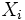$X_i$ is a connected space, but the product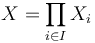$X = \prod_{i \in I} X_i$, endowed with the box topology, is not connected.

## Proof

Intuitively, the idea is that points that are far away from each other on infinitely many coordinates (as opposed to finitely many coordinates) are in different connected components.

### Proof using a countable power of the real line

Set each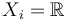$X_i = \R$ with the usual Euclidean space topology and suppose$I = \mathbb{N}$. Thus, the underlying set of$X$ is the set of all sequences of real numbers.$X$ can be represented as a union of two nonempty disjoint open subsets$U$ and$V$, where:

•$U$ is the set of bounded sequences, i.e., sequences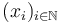$(x_i)_{i \in \mathbb{N}}$ for which there exists a real number$B$ satisfying$|x_i| \le B$ for all$i$.
•$V$ is the set of bounded sequences, i.e., sequences$(x_i)_{i \in \mathbb{N}}$ for which there exists no real number$B$ satisfying$|x_i| \le B$ for all$i$.

To see that both$U$ and$V$ are open, note that if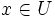$x \in U$, then all points in an open box of finite radius in all dimensions about$x$ is also in$U$. The corresponding statement is true for$V$.

Note that this proof crucially depends on the box topology, where it is possible to choose boxes that do not span the whole space in all (infinitely many) dimensions. These boxes would not be open in the product topology.